# SAT Math Multiple Choice Question 297: Answer and Explanation

### Test Information

Question: 297

12. Which of the following values satisfies the inequalities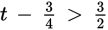and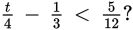• A. 1.75
• B. 2.25
• C. 2.75
• D. 3.25

Explanation:

C

Difficulty: Medium

Category: Heart of Algebra / Inequalities

Strategic Advice: Although the answer choices are given in decimal form, don't convert the fractions in the inequalities to decimals-this will create very messy calculations.

Getting to the Answer: Solve each inequality for t by first clearing all the fractions.

 First inequality: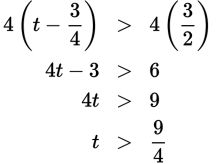Second inequality: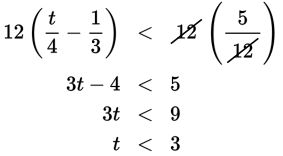Now, because the answer choices are given in decimal form, convert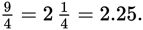The correct answer is greater (but not equal) to 2.25 and less than 3. The only answer that falls within this range is 2.75, making (C) correct.